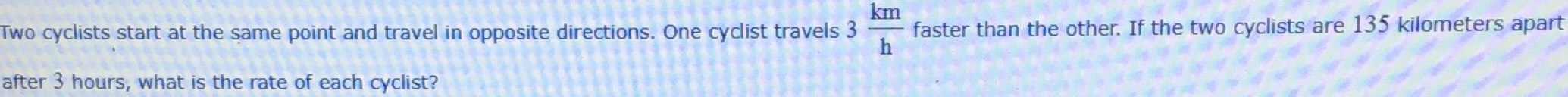¿Todavía tienes preguntas de matemáticas?

Pregunte a nuestros tutores expertos
Algebra
QuestionTwo cyclists start at the same point and travel in opposite directions. One cyclist travels $$3\mathrm{km/h}$$ faster than the other. If the two cyclists are $$135$$ kilometers apart after $$3$$ hours, what is the the rate of each cyclist?

$$21\mathrm{km/h}$$ and $$24\mathrm{km/h}$$